# Recreating a Geometric CDF plot from Casella and Berger

For reasons I no longer remember I decided a while back to reproduce Figure 1.5.2 from Casella and Berger (page 32):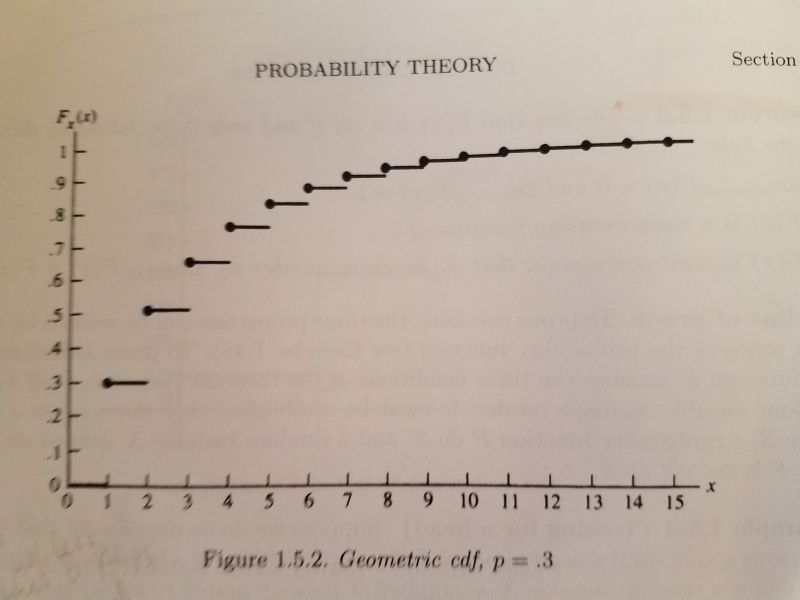It's a plot of the cumulative distribution function of a geometric distribution with p = 0.3. The geometric distribution is best explained with coin-flipping. Let's say we keep flipping a coin until we observe a head. Now let X be a random variable that equals the number of tosses required to see a head. So if we get a head on the first flip of the coin, that means we needed one toss. If it takes two tosses, we got a tail on the first toss and then a head on the second toss. The graph above visualizes the possibilities for 15 flips with probability of getting heads set to 0.3. We see that the probability of taking only one toss is 0.3, which makes sense since the probability of getting heads is 0.3. The probability of requiring two tosses is about 0.5. And so on. The straight lines indicate the probabilities only change at the whole numbers. There is no such thing as, say, 2.23 flips. This is often referred to as a step function. We see that there's a high probability of getting a head by the 5th or 6th flip. This is a simple waiting-time distribution that can be used to model the number of successes before a failure and vice-versa.

To recreate this plot in R I used the pgeom function, which returns the cumulative probability of the geometric distribution for a given number of failures before a success occurs and a given probability of success. For example, the probability of 0 failures before observing a success with p = 0.3:

pgeom(0, prob = 0.3)

##  0.3


The probability of 1 failure before observing a success with p = 0.3:

pgeom(1, prob = 0.3)

##  0.51


To generate all the probabilities in the plot, we can do the following:

y <- pgeom(q = 0:14, prob = 0.3)


Notice that instead of using 1:15 (number of total flips for success), we use 0:14. That's because pgeom works with the number of failures before success. Now that we have our y coordinates, we can create our first version of the plot as follows:

plot(x = 1:15, y, pch = 19)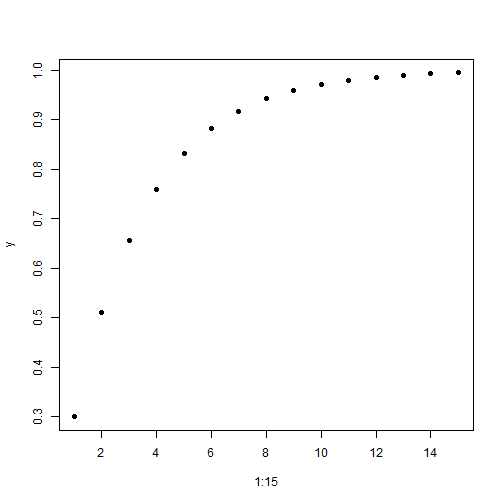Now let's add the horizontal lines using the segments function:

plot(x = 1:15, y, pch = 19)
segments(x0 = 0:15, y0 = c(0, y),
x1 = 1:16, y1 = c(0, y), lwd = 2)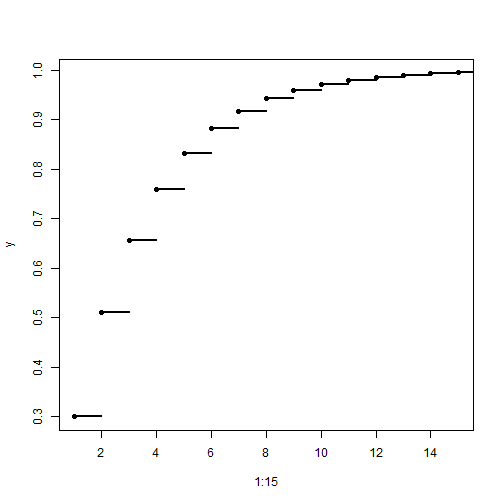The x0 and y0 coordinates are where the line starts. The x1 and y1 coordinates are where the line ends. Since the lines are horizontal, the y coordinates are the same for the start and end postions. The y coordinates include 0, so we add that value to y with the c function. The lwd = 2 argument makes the line a little thicker and darker.

Our plot is basically done, but just for fun I wanted to see how close I could make it look like the version in the book. That means relabeling the axes, moving the axis labels to the ends, and removing the lines at the top and right side of the plot. It also means moving the axis tick marks inside the plotting area. After some trial and error and deep dives into R documentation, here's what I was able to come up with:

plot(x = 1:15, y, pch = 19,
yaxt = "n", xaxt = "n", ylim = c(0,1.05), xlim = c(0,15.5),
bty="l", xaxs="i", yaxs = "i", xlab = "", ylab = "")
segments(x0 = 0:15, y0 = c(0, y),
x1 = 1:16, y1 = c(0, y), lwd = 2)
axis(side = 1, at = 0:15,
labels = 0:15, tcl = 0.5, family = "serif")
axis(side = 2, at = seq(0,1,0.1),
labels = c(0,paste(".",1:9),1), las=1, tcl = 0.5, family = "serif")
mtext(text = expression(italic(x)), side = 4,
at = 0, las = 1, line = 0.5, family = "serif")
mtext(text = expression(italic(F[x](x))), side = 3,
at = 0, line = 0.5, family = "serif")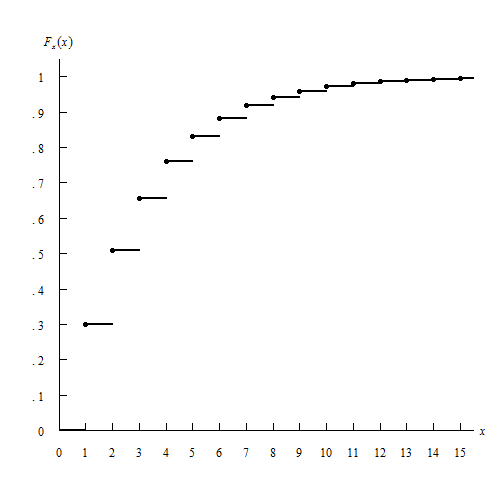In the plot function:

• the yaxt = "n" and xaxt = "n" arguments say “don't label the axes”. I instead use the axis function to create the axes.
• the ylim = c(0,1.05) and xlim = c(0,15.5) arguments tell the axes to end at 1.05 and 15.5, respectively. I wanted them to extend beyond the last value just as they do in the book.
• the bty="l" argument says “draw a box around the plot like a capital letter L”
• the xaxs="i" and yaxs = "i" arguments ensures the axes fit within the original range of the data. The default is to extend the range by 4 percent at each end. Again, I was trying to recreate the graph in the book. Notice how the origin has the x-axis and y-axis 0 values right next to one another.
• The xlab = "" and ylab = "" set blank axis labels. I instead use the mtext function to add axis labels.

The segments function remained unchanged.

The axis function allows us to explicitly define how the axis is created.

• The side argument specifies which side of the plot we're placing the axis. 1 is the bottom, 2 is the left.
• at is where we draw the tick marks.
• labels are how we label the tick marks.
• The tcl argument specifies how long to make the tick marks and in what direction. A positive value extends the tick marks into the plotting region.
• The las argument in the second axis function makes the labels on the y-axis horizontal.

Finally I used the mtext function to create the axis labels. mtext writes text into the margins of a plot and can take some trial and error to get the placement of text just so.

• The text argument is what text we want to place in the graph. In this case I make use of the expression function which allows us to create mathematical notation. For example, the syntax expression(italic(F[x](x))) returns $$F_x (x)$$
• The side argument again refers to where in the plot to place the text. 3 is top and 4 is right. This means the y-axis label is actually in the top of the plot and the x-axis label is on the right. A little bit of a hack.
• at says where to place the text along the axis parallel to the margin. In both cases we use 0. We want the y-axis label at the 0 point corresponding to the x-axis, and the x-axis label at the 0 point corresponding to the y-axis. A little confusing, I think.
• The las argument rotates the x label to be horizontal
• The line argument specifies on which margin line to place the text, starting at 0 counting outwards. This is one that takes some trial and error to get just right.
• The family argument specifies the type of font to use. “serif” is like Times New Roman.

Not perfect, but close enough. Of course I much prefer the R defaults when it comes to plotting layout. Even though R allows us to recreate this graph, I don't think it's necessarily a “good” graph.

I also decided to tackle this using ggplot. Here's how far I got.

library(ggplot2)
dat <- data.frame(x = 1:15, y = pgeom(q = 0:14, prob = 0.3))
dat2 <- data.frame(x = 0:15, y = c(0, dat$y[-16]), xend = 1:16, yend = c(0,dat$y[-16]))

ggplot(dat, aes(x = x, y = y)) + geom_point() +
geom_segment(aes(x = x, y = y, xend = xend, yend = yend), data = dat2) +
scale_x_continuous(breaks = 0:15, labels = 0:15) +
scale_y_continuous(breaks = seq(0,1,0.1), labels = c(0,paste(".",1:9),1)) +
labs(x = "x", y = expression(italic(F[x](x)))) +
theme(panel.background = element_blank(),
axis.line.x = element_line(color = "black"),
axis.line.y = element_line(color = "black"),
axis.title.x = element_text(face = "italic", hjust = 1),
axis.title.y = element_text(face = "italic", hjust = 1, angle = 0))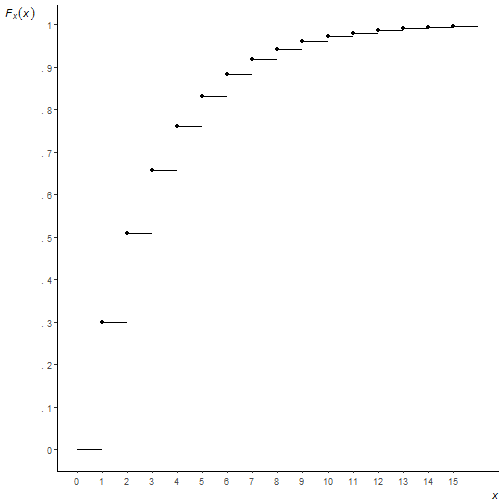You can see I couldn't figure out how to move the axis ticks into the plotting region, or how to place axis labels at the ends of the axis, or how to get the origin to start at precisely (0, 0). I'm not saying it can't be done, just that I lost interest in trying to go further. And a very strong argument can be made that these are things you shouldn't do anyway! But as I said at the outset, this was all for fun.

# Earthquake data and Benford’s Law

Much has been written about Benford's Law, that weird phenonmenon where if you have a naturally occuring set of numerical data, 30% of the numbers will begin with 1, 18% will begin with 2, 12% will begin with 3, and so on. You might expect the distribution of leading digits to be uniformly distributed, but no, that just isn't the case. 60% of the time the leading digit is 1, 2, or 3. Though it may seem like some curious mathematical anomaly, Benford's Law has been used to detect fraud in elections and accounting.

In this post let's use R to verify that earthquake data obtained from the USGS does indeed follow Benford's Law. On May 28, 2016, I downloaded data for all earthquakes in the past 30 days. The data has a number of fields, including earthquake magnitude, depth of the earthquake, location, time and many others. Benford's Law says those numbers should follow a particular distribution.

The formula for Benford's Law is as follows:

$P(d) = \log_{10} \left(1+\frac{1}{d}\right)$

That says the probability that a digit d occurs as the first number is equal the log base 10 of 1 + 1/d. We can quickly generate these for d = 1 – 9 in R:

log10(1 + (1/(1:9)))

##  0.30103000 0.17609126 0.12493874 0.09691001 0.07918125 0.06694679
##  0.05799195 0.05115252 0.04575749


And we can make a quick plot as follows:

barplot(log10(1 + (1/(1:9))), names.arg = 1:9, main = "Benford's Law")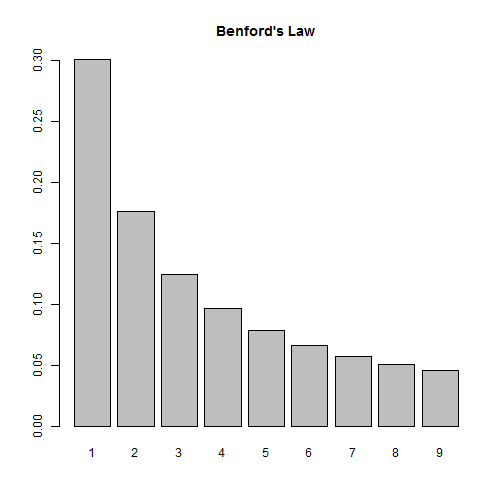So according to this law, if we look at the distribution of first digits in our earthquake data, we should see them closely follow this distribution. Let's find out!

First we need to import the data. Thanks to the USGS, this data comes ready for analysis. All we need to do is read it into R:

dat <- read.csv("all_month.csv")
nrow(dat)

##  8584


Over 8500 earthquakes in the past 30 days! A quick look at the magnitude shows us most of them are very small:

summary(dat$mag)  ## Min. 1st Qu. Median Mean 3rd Qu. Max. NA's ## -1.900 0.790 1.280 1.526 1.930 7.200 20  This also tells us we have some negative numbers (?) as well as some missing data, NA's. We also have numbers that start with 0 and have a decimal, such as 0.79. (In this case, Benford would say the number “starts” with a 7, not a 0.) This means when we determine the leading digits, we'll need to ignore negative signs, leading 0s, decimals and missing values. Let's investigate at the mag column. First we remove the NA's. Below is.na(dat$mag) generates a logical vector of TRUE and FALSE values. Adding an ! in front reverses the TRUE and FALSE values. Finally inserting the result in subsetting brackets returns only those values that are TRUE, ie not missing.

digits <- dat$mag[!is.na(dat$mag)]


Next we extract the first digits. To help with this I use two R packages, magrittr and stringr. The magrittr package allows us to “chain” commands together with the %>% operator. The stringr package provides the str_extract function that allows us to extract phrases that follow a certain pattern. So what we have below can be translated as follows:

• take the absoluet value of digits (to get rid of negative signs)
• convert digits to character (so we can use the next two functions)
• extract anything that is not a “0” or a “.” (We express this a regular expression: "[^0\\.]")
• extract the first digit (starting and ending at position 1)
library(magrittr)
library(stringr)
digits <- abs(digits) %>%
as.character() %>%
str_extract(pattern = "[^0\\.]") %>%
substr(1,1)


As an extra precaution, we then convert digits to a factor and set levels to be all digits 1 through 9. This ensure all digits are represented in subsequent calculations.

digits <- factor(digits, levels = 1:9) # ensure all digits represented


And finally we tally the first digits and calculate proportions:

table(digits) %>% prop.table()

## digits
##          1          2          3          4          5          6
## 0.42800141 0.17134139 0.05738763 0.09447248 0.05809177 0.03990142
##          7          8          9
## 0.04459570 0.05304542 0.05316277


We see 1 appearing a lot more often, but it's hard to tell how the other digits compare to Benford's Law. Let's put both distributions on the same graph. To do this, I went ahead and created a function that will allow us to check any vector of numbers against Benford's Law. Let's load the function and see how it works, then we'll break it down and explain it.

library(ggplot2)
compareBenford <- function(d){
digits <- d[!is.na(d)]
digits <- substr(stringr::str_extract(as.character(abs(digits)), pattern = "[^0\\.]"),1,1)
digits <- factor(digits, levels = 1:9) # ensure all digits represented
depth <- prop.table(table(digits))
ben <- log10(1 + (1/(1:9)))
dat2 <- data.frame(ben, depth)
names(dat2) <- c("Benford","Digit",deparse(substitute(d)))
dat2L <- reshape2::melt(dat2,id.vars="Digit", variable.name = "Type", value.name = "Frequency")
ggplot(dat2L, aes(x=Digit, y=Frequency, fill=Type)) +
geom_bar(stat = "identity", position = "dodge")
}

compareBenford(dat$mag)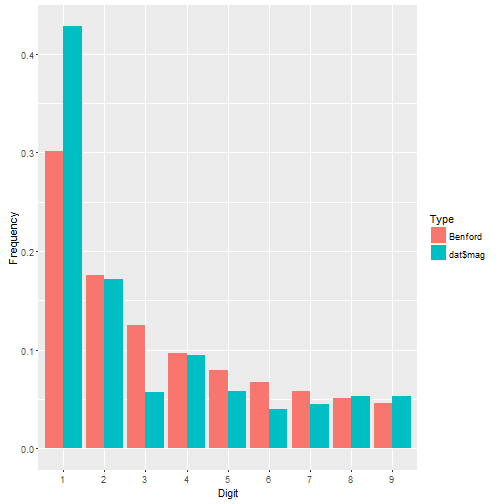We see dat$mag has more 1's than we might expect and fewer 3's, but otherwise seems to follow the distribution pretty closely. Let's check out earthquake depth.

compareBenford(dat$depth)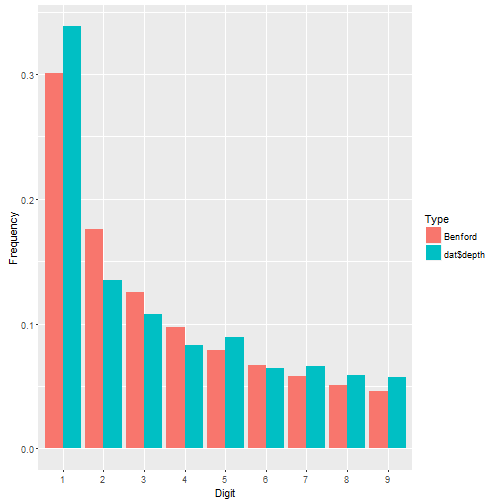This appears to fit even better. About the function: • the first four lines are what we did before. Notice I went ahead and nested the functions instead of using magrittr. That's how I originally coded it before deciding to write a blog post. Then I decided to break out magrittr for the fun of it. • After that I calculate Benford's proportions. • Then I put both sets of proportions in a data frame. • Then I change the names of the data frame. Notice there are three names to change, not two. Why? The depth vector is actually a table. When it gets pulled into a data frame, two columns are produced: the table cells and the table names. The names are the digits 1 – 9. Notice I also use deparse(substitute(d)) to name the first-digit proportions in the data frame. This ensures that whatever vector I give my function, the name of it will be the vector itself. So if I give it dat$mag, the column name of the first-digit proportions will be dat\$mag.
• Next I reshape the data using the melt function in the reshape2 package. This puts all the proportions in one column called Frequency and the type of proportion in another column called Type. I still have my Digit column that indicates which proportion goes with which digit. Having my data in this form allows me to use ggplot to map fill color to Type.
• Finally I create the graph. I set Digit on the x-axis, Frequency on the y-axis, and map Type to the fill color. The geom_bar function says draw a bar plot. The stat = "identity" and position = "dodge" arguments say set the height of the bars to the actual values and “dodge” them so they're next to one another.

Let's look at ALL numeric columns in the earthquakes data. We need to identify all numeric columns and pull them into a vector for our function to work. Here's how I do it:

theCols <- sapply(dat, class) %in% c("numeric","integer")
numCols <- names(dat)[theCols]
allDigits <- unlist(dat[,numCols])

compareBenford(allDigits)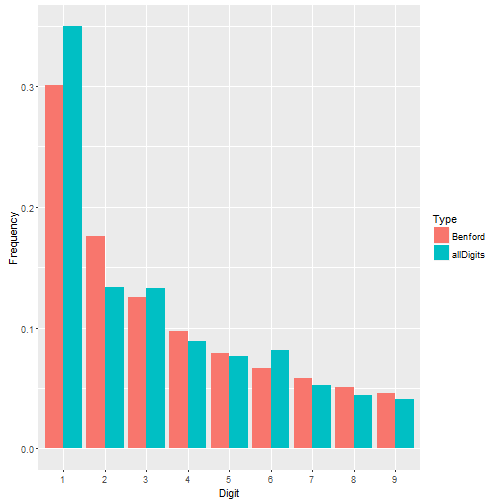Not a bad fit. Benford's Law seems to work just fine for the earthquake data.

But what about data where Benford's Law doesn't work? Recall that Benford's Law applies to most naturally occuring sets of numbers. Not all. Take the iris data that come with R. These are measurements of 50 flowers from each of 3 species of iris. This is a small data set looking at very specific measurements. It doesn't seem to follow Benford's Law very well.

irisDigits <- unlist(iris[,1:4])
compareBenford(irisDigits)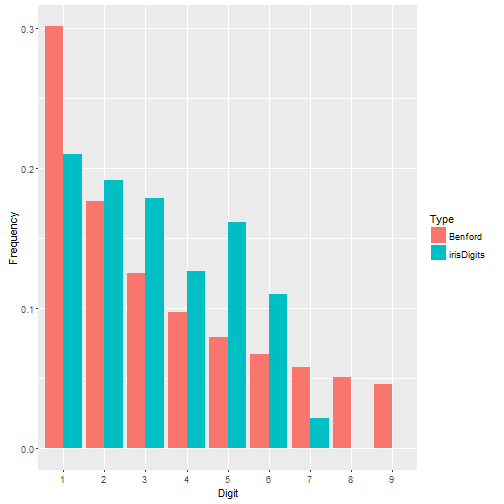# A Probability Problem in Heredity – Part 2

In my last post I solved a problem from chapter 2 of M.G. Bulmer’s Principles of Statistics. In this post I work through a problem in chapter 11 that is basically a continuation of the chapter 2 problem. If you take a look at the previous post, you’ll notice we were asked to find probability in terms of theta. I did it and that’s nice and all, but we can go further. We actually have data, so we can estimate theta. And that’s what the problem in chapter 11 asks us to do. If you’re wondering why it took 9 chapters to get from finding theta to estimating theta, that’s because the first problem involved basic probability and this one requires maximum likelihood. It’s a bit of a jump where statistical background is concerned.

The results of the last post were as follows:

 purple-flowered red-flowered long pollen $$\frac{1}{4}(\theta + 2)$$ $$\frac{1}{4}(1 – \theta)$$ round pollen $$\frac{1}{4}(1 – \theta)$$ $$\frac{1}{4}\theta$$

The table provides probabilities of four possible phenotypes when hybrid sweet peas are allowed to self-fertilize. For example, the probability of a self-fertilizing sweet pea producing a purple-flower with long pollen is $$\frac{1}{4}(1 – \theta)$$. In this post we’ll estimate theta from our data. Recall that $$\theta = (1 – \pi)^{2}$$, where $$\pi$$ is the probability of the dominant and recessive genes of a characteristic switching chromosomes.

Here’s the data:

 Purple-flowered Red-flowered Long pollen 1528 117 Round pollen 106 381

We see from the table there are 4 exclusive possibilities when the sweet pea self-fertilizes. If we think of each possibility having its own probability of occurrence, then we can think of this data as a sample from a multinomial distribution. Since chapter 11 covers maximum likelihood estimation, the problem therefore asks us to use the multinomial likelihood function to estimate theta.

Now the maximum likelihood estimator for each probability is $$\hat{p_{i}} = \frac{x_{i}}{n}$$. But we can’t use that. That’s estimating four parameters. We need to estimate one parameter, theta. So we need to go back to the multinomial maximum likelihood function and define $$p_{i}$$ in terms of theta. And of course we’ll work with the log likelihood since it’s easier to work with sums than products.

$$\log L(\theta) = y_{1} \log p_{1} + y_{2} \log p_{2} + y_{3} \log p_{3} + y_{4} \log p_{4}$$

If you’re not sure how I got that, google “log likelihood multinomial distribution” for more PDF lecture notes than you can ever hope to read.

Now let’s define the probabilities in terms of one parameter, theta:

$$\log L(\theta) = y_{1} \log f_{1}(\theta) + y_{2} \log f_{2}(\theta) + y_{3} \log f_{3}(\theta) + y_{4} \log f_{4}(\theta)$$

Now take the derivative. Once we have that we can set equal to 0 and find a solution for theta. The solution will be the point at which theta obtains its maximum value:

$$\frac{d \log L(\theta)}{d\theta} = \frac{y_{1}}{f_{1}(\theta)} f’_{1}(\theta) + \frac{y_{1}}{f_{2}(\theta)} f’_{2}(\theta) + \frac{y_{1}}{f_{3}(\theta)} f’_{3}(\theta) + \frac{y_{1}}{f_{4}(\theta)} f’_{4}(\theta)$$

Time to go from the abstract to the applied with our values. The y’s are the data from our table and the functions of theta are the results from the previous problem.

$$\frac{d \log L(\theta)}{d\theta} = \frac{1528}{1/4(2 + \theta)} \frac{1}{4} – \frac{117}{1/4(1 – \theta)}\frac{1}{4} – \frac{106}{1/4(1 – \theta)}\frac{1}{4} + \frac{381}{1/4(\theta)} \frac{1}{4}$$
$$\frac{d \log L(\theta)}{d\theta} = \frac{1528}{2 + \theta} – \frac{117}{1 – \theta} – \frac{106}{1 – \theta} + \frac{381}{\theta}$$
$$\frac{d \log L(\theta)}{d\theta} = \frac{1528}{2 + \theta} – \frac{223}{1 – \theta} + \frac{381}{\theta}$$

Set equal to 0 and solve for theta. Beware, it gets messy.

$$\frac{[1528(1 – \theta)\theta] – [223(2 + \theta)\theta] + [381(2 + \theta)(1 – \theta)]}{(2 + \theta)(1- \theta)\theta} = 0$$

Yeesh. Fortunately the denominator cancels out when we start multiplying terms and solving for theta. So we’re left with this:

$$1528\theta – 1528\theta^{2} – 446\theta – 223\theta^{2} + 762 – 381\theta – 381\theta^{2} = 0$$

And that reduces to the following quadratic equation:

$$-2132\theta^{2} + 701\theta + 762 = 0$$

I propose we use an online calculator to solve this equation. Here’s a good one. Just plug in the coefficients and hit solve to find the roots. Our coefficients are a = -2132, b = 701, and c = 762. Since it’s a quadratic equation we get two answers:

$$x_{1} = -0.4556$$
$$x_{2} = 0.7844$$

The answer is in terms of a probability which is between 0 and 1, so we toss the negative answer and behold our maximum likelihood estimator for theta: 0.7844.

Remember that $$\theta = (1 – \pi)^{2}$$. If we solve for pi, we get $$\pi = 1 – \theta^{1/2}$$, which works out to be 0.1143. That is, we estimate the probability of characteristic genes switching chromosomes to be about 11%. Therefore we can think of theta as the probability of having two parents that experienced no gene switching.

Now point estimates are just estimates. We would like to know how good the estimate is. That’s where confidence intervals come in to play. Let’s calculate one for theta.

It turns out that we can estimate the variability of our maximum likelihood estimator as follows:

$$V(\theta) = \frac{1}{I(\theta)}$$, where $$I(\theta) = -E(\frac{d^{2}\log L}{d \theta^{2}})$$

We need to determine the second derivative of our log likelihood equation, take the expected value by plugging in our maximum likelihood estimator, multiply that by -1, and then take the reciprocal. The second derivative works out to be:

$$\frac{d^{2}\log L}{d \theta^{2}} = -\frac{1528}{(2 + \theta)^{2}} -\frac{223}{(1 – \theta)^{2}} -\frac{381}{\theta^{2}}$$

The negative expected value of the second derivative is obtained by plugging in our estimate of 0.7844 and multiplying by -1. Let’s head to the R console to calculate this:

th <- 0.7844 # our ML estimate
(I <- -1 * (-1528/((2+th)^2) - 223/((1-th)^2) - 381/(th^2))) # information
 5613.731


Now take the reciprocal and we have our variance:

(v.th <- 1/I)
 0.0001781347


We can take the square root of the variance to get the standard error and multiply by 1.96 to get the margin of error for our estimate. Then add/subtract the margin of error to our estimate for a confidence interval:

# confidence limits on theta
0.784+(1.96*sqrt(v.th)) # upper bound
 0.8101596
0.784-(1.96*sqrt(v.th)) # lower bound
 0.7578404


Finally we convert the confidence interval for theta to a confidence interval for pi:

# probability of switching over
th.ub <- 0.784+(1.96*sqrt(v.th))
th.lb <- 0.784-(1.96*sqrt(v.th))
1-sqrt(th.ub) # lower bound
 0.09991136
1-sqrt(th.lb) # upper bound
 0.1294597


The probability of genes switching chromosomes is most probably in the range of 10% to 13%.

# Deriving the Poisson Distribution

The Poisson distribution can be derived from the binomial distribution by doing two steps:

1. substitute$\frac{\mu}{n}$ for p
2. Let n increase without bound

Step one is possible because the mean of a binomial distribution is$\mu = np$. So another way of expressing p, the probability of success on a single trial, is$\frac{\mu}{n}$. This has some intuition. Recall that a binomial distribution gives the probability of a number of successes (x) in a fixed number of trials (n) for some probability (p). So if an event has a probability of 0.2, and we observe 10 trials (where the trials are independent), the expected value of seeing the event occur is 10(0.2) = 2. On average we would see the event happen twice in 10 trials. Which means we can state the probability as a rate,$\frac{2}{10}$. Success occurs, on average, 2 times per 10 trials.

The next step leads to the Poisson. We let the number of trials increase without bound, which means the probability shrinks. Since n is in the denominator of$\frac{\mu}{n}$, this gives a small chance of success in a huge number of trials. So whereas the binomial deals with a fixed number of trials, the Poisson accounts for an unlimited number of trials in time or space. Taking the limit as n tends to infinity gives us the Poisson. Here’s how.

Recall the binomial probability mass function:$f(x) = \frac{n!}{x!(n-x)!}p^{x}(1-p)^{n-x}$

Substitute$\frac{\mu}{n}$ for p:$f(x) = \frac{n!}{x!(n-x)!}(\frac{\mu}{n})^{x}(1-\frac{\mu}{n})^{n-x}$

Now the fun begins. Let n grow very large:$\lim_{n \to \infty}\frac{n!}{x!(n-x)!}(\frac{\mu}{n})^{x}(1-\frac{\mu}{n})^{n-x}$

But wait! Don’t let n blow up just yet. Let’s do some rearranging first to help us take the limit:$\lim_{n \to \infty}\frac{n!}{(n-x)!n^{x}}\frac{\mu^{x}}{x!}(1-\frac{\mu}{n})^{n}(1-\frac{\mu}{n})^{-x}$

And let’s also simplify$\frac{n!}{(n-x)!n^{x}}$:$\frac{n!}{(n-x)!n^{x}} = \frac{n(n-1)(n-2)\dots(n-x+1)}{n^{x}}$$=1(1-\frac{1}{n})\dots(1-\frac{x-2}{n})(1-\frac{x-1}{n})$

Before we flip the switch on the limit-to-infinity machine, let’s assess what we got:$\lim_{n \to \infty}1(1-\frac{1}{n})\dots(1-\frac{x-2}{n})(1-\frac{x-1}{n})\frac{\mu^{x}}{x!}(1-\frac{\mu}{n})^{n}(1-\frac{\mu}{n})^{-x}$

Notice this is basically four things multiplied together:

1.$1(1-\frac{1}{n})\dots(1-\frac{x-2}{n})(1-\frac{x-1}{n})$
2.$\frac{\mu^{x}}{x!}$
3.$(1-\frac{\mu}{n})^{n}$
4.$(1-\frac{\mu}{n})^{-x}$

We can make life easier by taking the limit of each of those four pieces individually:

1.$\lim_{n \to \infty}1(1-\frac{1}{n})\dots(1-\frac{x-2}{n})(1-\frac{x-1}{n})=1$
2.$\lim_{n \to \infty}\frac{\mu^{x}}{x!}=\frac{\mu^{x}}{x!}$ (no n to blow up!)
3.$\lim_{n \to \infty}(1-\frac{\mu}{n})^{n}=e^{-\mu}$
4.$\lim_{n \to \infty}(1-\frac{\mu}{n})^{-x}=1$

1, 2, and 4 are easy. Number 3, though, requires a long forgotten formula you probably learned in calculus just long enough to take an exam:$\lim_{n \to \infty}(1+\frac{b}{n})^{n}=e^{b}$

Set b =$-\mu$ and you get the result.

Now put it all back together and you have the probability mass function of the Poisson distribution:$f(x) = \frac{\mu^{x}e^{-\mu}}{x!}$

Often you see$\mu$ as$\lambda$ in this formula.

This new formula is approximately the binomial probability mass function for a large number of trials and small probability. That’s basically what we did, right? Substitute an expression for p involving n and let n grow large. That means the probability got small while n got large. Which further means that before we had modern computers, the Poisson was very handy for approximating binomial probabilities since the binomial coefficient can be difficult to compute for large n.

For example, say we have 100 independent trials with probability of success 0.05. What is the probability of observing 7 successes?

Using the binomial:$f(7) = \frac{100!}{7!(100-7)!}0.05^{7}(1-0.05)^{100-7} = 0.106026$

Using the Poisson as an approximation (where$\mu = np = 100(0.05) = 5$):$f(7) = \frac{5^{7}e^{-5}}{7!} = 0.104445$

Very close indeed!

By the way, how did I calculate those probabilities? I used Excel functions:

Binomial: =BINOMDIST(7,100,0.05,0)
Poisson: =POISSON(7,5,0)

The numbers in the parentheses should be self-explanatory. The final 0 means “false, no cumulative”. If you set it to 1, you get the probability of 7 or fewer successes in 100 trials. You can also use a TI-83 Plus. Just press 2nd – DISTR and choose binompdf or poissonpdf. If want to do cumulative probabilities, select binomcdf or poissoncdf. The parameters are in a different order from Excel:

binomcdf/binompdf(n,p,x)
poissoncdf/poissonpdf(mu,x)

Of course “real statisticians” would use R:

> dbinom(7,100,0.05)
 0.1060255

> dpois(7,5)
 0.1044449

I would be careless not to mention that the Poisson distribution is not the same as the binomial. The binomial describes the distribution of events (or “successes”) over a fixed number of trials for a fixed probability of success on each trial. The Poisson describes the distribution of events in space or time for a given rate of occurrence. The binomial is specified by n (number of trails) and p (probability of success on each trial). The Poisson is specified by$\mu$, the rate at which events occur per unit of space or time.

And finally, and no doubt most important, the way to pronounce Poisson is \pwa-sawn\. Say it correctly and you earn instant credibility at cocktail parties.

# How many rolls of a die to see all sides at least once?

Say you have a fair die. (Most of them are these days.) You roll it once and observe a 3. You roll it again and observe a 1. You roll it yet again and get a 4. In three throws you’ve observed 3 of the possible sides. What if you keep going? How many rolls would it take to see all six sides at least once. Obviously the minimum number is 6. But the probability of that happening is about 0.015. ($1 \times \frac{5}{6}\times \frac{4}{6}\times \frac{3}{6}\times \frac{2}{6}\times \frac{1}{6}$). What is the most likely number of rolls? Or put in the language of a statistics textbook, what’s the expected number of rolls?

To solve this problem we need to use the Geometric Distribution. If a series of trials are independent of one another, and each trial has the same probability of success, then the number of trials needed to observe the first success has a geometric distribution. This is the scenario of our roll of the die. Each roll is independent of one another and the probability of success remains the same for each roll, whatever we define “success” to be. The mean of a geometric distribution is$\frac{1}{p}$. That means the expected number of times we need to roll a dice to observe, say, a four is 6.

Back to our problem. We’re certain to get at least one of the faces on the first roll. So that probability is 1. On the second roll we have a probability of$\frac{5}{6}$ of getting a different face than what we got on the first roll. It’s not certain. There is a probability of$\frac{1}{6}$ of getting the same result as the first roll. Once we have observed two sides, the probability of observing a third side becomes$\frac{4}{6}$. On so on. Notice that as we observe previously unseen sides the definition of success changes, therefore the probability changes. So to determine the expected number of rolls to see all sides of a die at least once, we need to find the expected number of rolls for each definition of “success” and sum them. The steps below describe the process. (The numbers represent the order of the observed sides, not the values on the dice.)

1. We’re guaranteed to see one of the sides on the first roll. Expected number of rolls: 1
2. We have$\frac{5}{6}$ probability of seeing a different side than what was previously observed. Expected number of rolls:$1/\frac{5}{6}=1.2$
3. We have$\frac{4}{6}$ probability of seeing a different side than what was previously observed in steps 1 and 2. Expected number of rolls:$1/\frac{4}{6}=1.5$
4. We have$\frac{3}{6}$ probability of seeing a different side than what was previously observed in steps 1 -3. Expected number of rolls:$1/\frac{3}{6}=2$
5. We have$\frac{2}{6}$ probability of seeing a different side than what was previously observed in steps 1-4. Expected number of rolls:$1/\frac{2}{6}=3$
6. We have$\frac{1}{6}$ probability of seeing a different side than what was previously observed in steps 1-5. Expected number of rolls:$1/\frac{1}{6}=6$

Adding all the expected number of rolls for each definition of success we get 14.7. So we expect to roll a die about 15 times on averageÂ  before we observe all sides at least once.

We can use this same line of thinking to answer such “real-life” questions as how many boxes of cereal would you have to buy to collect the latest series of cheap plastic toys, assuming the toys are randomly added to the cereal in a uniform manner. Of course if you’re desperate to collect the precious toys and have a modicum of patience, the answer is probably 0. You’d just wait to buy the whole set on eBay.